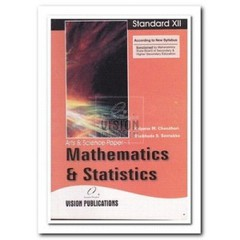# MATHEMATICS & STATISTICS P-I

(Inclusive of all Taxes)

Rs. 47

## Details

Contents

5. Parabola, Ellipse and Hyperbola
5.1 Introduction
5.2 Conic sections
5.3 Parabola
5.4 Introduction
6. Vectors
6.1 Introduction
6.2 Collinear vectors
6.3 Coplanar Vectors
6.4 Linear combination of the vectors
6.5 Position vector of a point
6.6 Section formula
6.7 Midpoint and centroid formula
6.8 Scalar - triple - product or Box product of the vectors
6.9 Geometrical meaning of
6.10 Sign of the box product
6.11 Volume of tetrahedron
7. Applications of vectors
7.1 Introduction
8. Line
8.1 Introduction
8.2 Direction angles and direction cosines
8.3 Line
9. Plane
9.1 Introduction
9.2 Equation of plane
10. Linear Programming
10.1 Introduction
10.2 Some terms related to linear programming Problem (L.P.P)
10.3 Linear Programming Problem
10.4 Formulation of a linear programming problem
10.5 Problem solving
10.6 Simplex Method
11. Probability
11.1 Introduction
11.2 Terms related to Theory of Probability
11.3 Compound events
11.4 Conditional Probability and Multiplication Theorem
11.5 Inverse Probability - Bayes Theorem
11.6 Probability Distribution
11.7 Two Discrete Probability Distributions

• Phase Junior College

• Stream Arts,Science

• Standard/Year 12th

• Medium English

• Board/University Maharashtra State Board

• Subject Mathematics

#### Reviews

• No Reviews written for this product. Be the first to write a review.